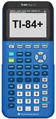Factoring - Algebraic Examples MathBitsNotebook.com Terms of Use   Contact Person: Donna RobertsThe greatest common factor (GCF) of two (or more) monomials is the product of the greatest common factor of the numerical coefficients (the numbers out in front) and the highest power of every variable that is a factor of each monomial.Consider: 10x2y3 and 15xy2   The greatest common factor is 5xy2 . The largest factor of 10 and 15 is 5. The highest power of x that is contained in both terms is x. The highest power of y that is contained in both terms is y2.

When factoring polynomials, first look for the largest monomial which is a factor of each term of the polynomial.   Factor out (divide each term by) this largest monomial.

 Factor:  4x + 8y The largest integer that will divide evenly into 4 and 8 is 4.  Since the terms do not contain a variable (x or y) in common, we cannot factor any variables. The greatest common factor is 4.  Divide each term by 4. Answer:  4(x + 2y)Factor:  15x2y3 + 10xy2 The largest integer that divides evenly into 15 and 10 is 5.  The largest power of x present in both terms is x. The largest power of y present in both term is y2. The GCF is 5xy2.  Divide each term by the GCF. Answer:  5xy2(3xy + 2)See how to use your TI-83+/84+ graphing calculator  with factoring. Click calculator.